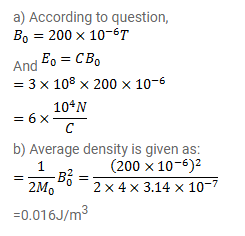# The magnetic field in a plane electromagnetic wave is given by

Question:

The magnetic field in a plane electromagnetic wave is given by

$B=(200 \mu T) \sin \left[\left(4.0 \times 10^{15} s^{-1}\right)(t-x / c)\right]$

Find the maximum electric field and the average energy density corresponding to the electric field.

Solution: